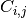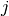# `sklearn.metrics`.confusion_matrix¶

`sklearn.metrics.``confusion_matrix`(y_true, y_pred, labels=None)[源代码]

Compute confusion matrix to evaluate the accuracy of a classification

By definition a confusion matrixis such thatis equal to the number of observations known to be in groupbut predicted to be in group.

Read more in the User Guide.

Parameters: y_true : array, shape = [n_samples] Ground truth (correct) target values. y_pred : array, shape = [n_samples] Estimated targets as returned by a classifier. labels : array, shape = [n_classes], optional List of labels to index the matrix. This may be used to reorder or select a subset of labels. If none is given, those that appear at least once in `y_true` or `y_pred` are used in sorted order. C : array, shape = [n_classes, n_classes] Confusion matrix

References

Examples

```>>> from sklearn.metrics import confusion_matrix
>>> y_true = [2, 0, 2, 2, 0, 1]
>>> y_pred = [0, 0, 2, 2, 0, 2]
>>> confusion_matrix(y_true, y_pred)
array([[2, 0, 0],
[0, 0, 1],
[1, 0, 2]])
```
```>>> y_true = ["cat", "ant", "cat", "cat", "ant", "bird"]
>>> y_pred = ["ant", "ant", "cat", "cat", "ant", "cat"]
>>> confusion_matrix(y_true, y_pred, labels=["ant", "bird", "cat"])
array([[2, 0, 0],
[0, 0, 1],
[1, 0, 2]])
```